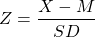# Worksheet 4a.

These guided exercises will give you the opportunity to practice the techniques and apply the concepts you learned in Chapter 4a. You will need some paper, spreadsheet, or a tablet to work on the analyses. For this exercise, you also need a coin!

1. A fair coin toss should theoretically result in heads 50% of the time. Suppose we want to find out if this is really true in our classroom. We will measure the number of times a coin comes up heads in 10 coin tosses.

d. Toss a coin 10 times and record the results. Count up the number of heads.

e. Find the probability associated with your sample number of heads if the population distribution of number of heads has a mean 5 and standard deviation is 1. To do this, first sketch a normal distribution and label with raw scores and z-scores. Convert your number of heads into a z-score. Shade in the probability of getting an outcome at least as extreme as the one you found on the normal curve you sketched. Find the area under the normal curve associated with the z-score of the number you found or more extreme. (Note that more extreme scores could be on either end of the distribution.)f. Given our usual threshold of two standard deviations away from the mean to define extreme scores, was the number of heads in your sample really surprising?